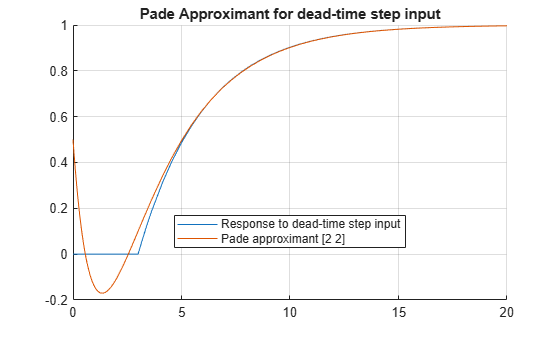The Padé approximant of order [mn] approximates the function f(x) around x = x0 as

`$\frac{{a}_{0}+{a}_{1}\left(x-{x}_{0}\right)+...+{a}_{m}{\left(x-{x}_{0}\right)}^{m}}{1+{b}_{1}\left(x-{x}_{0}\right)+...+{b}_{n}{\left(x-{x}_{0}\right)}^{n}}.$`

The Padé approximant is a rational function formed by a ratio of two power series. Because it is a rational function, it is more accurate than the Taylor series in approximating functions with poles. The Padé approximant is represented by the Symbolic Math Toolbox™ function `pade`.

When a pole or zero exists at the expansion point x = x0, the accuracy of the Padé approximant decreases. To increase accuracy, an alternative form of the Padé approximant can be used which is

`$\frac{{\left(x-{x}_{0}\right)}^{p}\left({a}_{0}+{a}_{1}\left(x-{x}_{0}\right)+...+{a}_{m}{\left(x-{x}_{0}\right)}^{m}\right)}{1+{b}_{1}\left(x-{x}_{0}\right)+...+{b}_{n}{\left(x-{x}_{0}\right)}^{n}}.$`

The `pade` function returns the alternative form of the Padé approximant when you set the `OrderMode` input argument to `Relative`.

The Padé approximant is used in control system theory to model time delays in the response of the system. Time delays arise in systems such as chemical and transport processes where there is a delay between the input and the system response. When these inputs are modeled, they are called dead-time inputs. This example shows how to use the Symbolic Math Toolbox to model the response of a first-order system to dead-time inputs using Padé approximants.

The behavior of a first-order system is described by this differential equation

`$\tau \frac{dy\left(t\right)}{dt}+y\left(t\right)=ax\left(t\right).$`

Enter the differential equation in MATLAB®.

```syms tau a x(t) y(t) xS(s) yS(s) H(s) tmp F = tau*diff(y)+y == a*x;```

Find the Laplace transform of `F` using `laplace`.

`F = laplace(F,t,s)`
`F = $\mathrm{laplace}\left(y\left(t\right),t,s\right)-\tau \left(y\left(0\right)-s \mathrm{laplace}\left(y\left(t\right),t,s\right)\right)=a \mathrm{laplace}\left(x\left(t\right),t,s\right)$`

Assume the response of the system at `t = 0` is `0`. Use `subs` to substitute for `y(0) = 0`.

`F = subs(F,y(0),0)`
`F = $\mathrm{laplace}\left(y\left(t\right),t,s\right)+s \tau \mathrm{laplace}\left(y\left(t\right),t,s\right)=a \mathrm{laplace}\left(x\left(t\right),t,s\right)$`

To collect common terms, use `simplify`.

`F = simplify(F)`
`F = $\left(s \tau +1\right) \mathrm{laplace}\left(y\left(t\right),t,s\right)=a \mathrm{laplace}\left(x\left(t\right),t,s\right)$`

For readability, replace the Laplace transforms of `x(t)` and `y(t)` with `xS(s)` and `yS(s)`.

`F = subs(F,[laplace(x(t),t,s) laplace(y(t),t,s)],[xS(s) yS(s)])`
`F = $\mathrm{yS}\left(s\right) \left(s \tau +1\right)=a \mathrm{xS}\left(s\right)$`

The Laplace transform of the transfer function is `yS(s)/xS(s)`. Divide both sides of the equation by `xS(s)` and use `subs` to replace `yS(s)/xS(s)` with `H(s)`.

```F = F/xS(s); F = subs(F,yS(s)/xS(s),H(s))```
`F = $H\left(s\right) \left(s \tau +1\right)=a$`

Solve the equation for `H(s)`. Substitute for `H(s)` with a dummy variable, solve for the dummy variable using `solve`, and assign the solution back to `H(s)`.

```F = subs(F,H(s),tmp); H(s) = solve(F,tmp)```
```H(s) =  $\frac{a}{s \tau +1}$```

The input to the first-order system is a time-delayed step input. To represent a step input, use `heaviside`. Delay the input by three time units. Find the Laplace transform using `laplace`.

```step = heaviside(t - 3); step = laplace(step)```
```step =  $\frac{{\mathrm{e}}^{-3 s}}{s}$```

Find the response of the system, which is the product of the transfer function and the input.

`y = H(s)*step`
```y =  $\frac{a {\mathrm{e}}^{-3 s}}{s \left(s \tau +1\right)}$```

To allow plotting of the response, set parameters `a` and `tau` to their values. For `a` and `tau`, choose values `1` and `3`, respectively.

```y = subs(y,[a tau],[1 3]); y = ilaplace(y,s);```

Find the Padé approximant of order `[2 2]` of the step input using the `Order` input argument to `pade`.

`stepPade22 = pade(step,'Order',[2 2])`
```stepPade22 =  $\frac{3 {s}^{2}-4 s+2}{2 s \left(s+1\right)}$```

Find the response to the input by multiplying the transfer function and the Padé approximant of the input.

`yPade22 = H(s)*stepPade22`
```yPade22 =  $\frac{a \left(3 {s}^{2}-4 s+2\right)}{2 s \left(s \tau +1\right) \left(s+1\right)}$```

Find the inverse Laplace transform of `yPade22` using `ilaplace`.

`yPade22 = ilaplace(yPade22,s)`
```yPade22 =  $a+\frac{9 a {\mathrm{e}}^{-s}}{2 \tau -2}-\frac{a {\mathrm{e}}^{-\frac{s}{\tau }} \left(2 {\tau }^{2}+4 \tau +3\right)}{\tau \left(2 \tau -2\right)}$```

To plot the response, set parameters `a` and `tau` to their values of `1` and `3`, respectively.

`yPade22 = subs(yPade22,[a tau],[1 3])`
```yPade22 =  $\frac{9 {\mathrm{e}}^{-s}}{4}-\frac{11 {\mathrm{e}}^{-\frac{s}{3}}}{4}+1$```

Plot the response of the system `y` and the response calculated from the Padé approximant `yPade22`.

```hold on grid on fplot([y yPade22],[0 20]) title('Pade Approximant for dead-time step input') legend('Response to dead-time step input',... 'Pade approximant [2 2]',... 'Location', 'Best')```The `[2 2]` Padé approximant does not represent the response well because a pole exists at the expansion point of `0`. To increase the accuracy of `pade` when there is a pole or zero at the expansion point, set the `OrderMode` input argument to `Relative` and repeat the steps. For details, see `pade`.

`stepPade22Rel = pade(step,'Order',[2 2],'OrderMode','Relative')`
```stepPade22Rel =  $\frac{3 {s}^{2}-6 s+4}{s \left(3 {s}^{2}+6 s+4\right)}$```
`yPade22Rel = H(s)*stepPade22Rel`
```yPade22Rel =  $\frac{a \left(3 {s}^{2}-6 s+4\right)}{s \left(s \tau +1\right) \left(3 {s}^{2}+6 s+4\right)}$```
`yPade22Rel = ilaplace(yPade22Rel)`
```yPade22Rel =  ```
`yPade22Rel = subs(yPade22Rel,[a tau],[1 3])`
```yPade22Rel =  $\frac{12 {\mathrm{e}}^{-t} \left(\mathrm{cos}\left(\frac{\sqrt{3} t}{3}\right)+\frac{2 \sqrt{3} \mathrm{sin}\left(\frac{\sqrt{3} t}{3}\right)}{3}\right)}{7}-\frac{19 {\mathrm{e}}^{-\frac{t}{3}}}{7}+1$```
`fplot(yPade22Rel,[0 20],'DisplayName','Relative Pade approximant [2 2]')`The accuracy of the Padé approximant can also be increased by increasing its order. Increase the order to `[4 5]` and repeat the steps. The `[n-1 n]` Padé approximant is better at approximating the response at `t = 0` than the `[n n]` Padé approximant.

`stepPade45 = pade(step,'Order',[4 5])`
```stepPade45 =  $\frac{27 {s}^{4}-180 {s}^{3}+540 {s}^{2}-840 s+560}{s \left(27 {s}^{4}+180 {s}^{3}+540 {s}^{2}+840 s+560\right)}$```
`yPade45 = H(s)*stepPade45`
```yPade45 =  $\frac{a \left(27 {s}^{4}-180 {s}^{3}+540 {s}^{2}-840 s+560\right)}{s \left(s \tau +1\right) \left(27 {s}^{4}+180 {s}^{3}+540 {s}^{2}+840 s+560\right)}$```
`yPade45 = subs(yPade45,[a tau],[1 3])`
```yPade45 =  $\frac{27 {s}^{4}-180 {s}^{3}+540 {s}^{2}-840 s+560}{s \left(3 s+1\right) \left(27 {s}^{4}+180 {s}^{3}+540 {s}^{2}+840 s+560\right)}$```
`yPade45 = ilaplace(yPade45)`
```yPade45 =  ```
`yPade45 = vpa(yPade45)`
`yPade45 = $3.2418384981662546679005910164486 {\mathrm{e}}^{-1.930807068546914778929595950184 t} \mathrm{cos}\left(0.57815608595633583454598214328008 t\right)-2.7182817182817182817182817182817 {\mathrm{e}}^{-0.33333333333333333333333333333333 t}-1.5235567798845363861823092981669 {\mathrm{e}}^{-1.4025262647864185544037373831494 t} \mathrm{cos}\left(1.7716120279045018112388813990878 t\right)+11.595342871672681856604670597166 {\mathrm{e}}^{-1.930807068546914778929595950184 t} \mathrm{sin}\left(0.57815608595633583454598214328008 t\right)-1.7803798379230333426855987436911 {\mathrm{e}}^{-1.4025262647864185544037373831494 t} \mathrm{sin}\left(1.7716120279045018112388813990878 t\right)+1.0$`
`fplot(yPade45,[0 20],'DisplayName','Pade approximant [4 5]')`The following points have been shown:

• When a pole or zero exists at the expansion point, the Padé approximant is inaccurate about the expansion point. To increase the accuracy of the approximant, set the `OrderMode` option to `Relative`. You can also use increase the order of the denominator relative to the numerator.